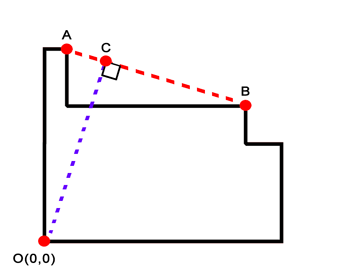Angled Width of a Sofa
View the Lesson | MATHguide homepage Updated June 22nd, 2023

Problem: Find the angled width of the sofa with the given dimensions.Dimensions
height = 30 in
width = 31 in
arm length = 27 in
arm height = 26 in
thickness = 4 inLocation of Points
A(,)
B(,)

Slope of Segment ABEquation of Line AB
 y = x +Slope of Segment COEquation of Line CO
 y = xLocation of C
C(,)

Length of Segment CO
in